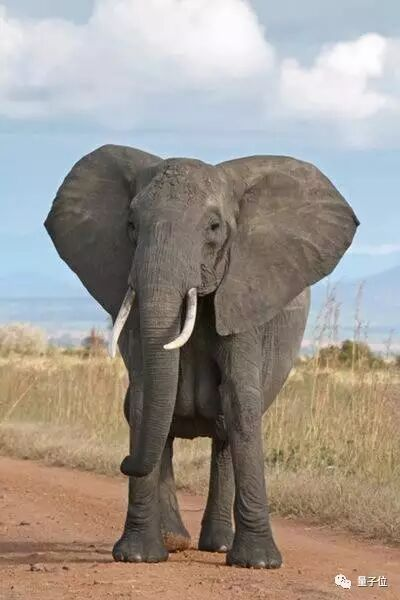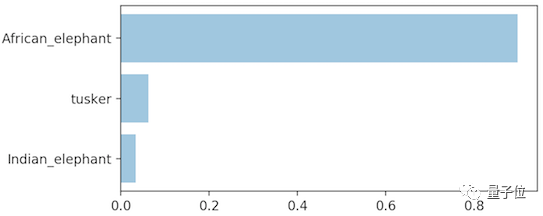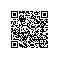# 用Keras+TensorFlow，实现ImageNet数据集日常对象的识别# Keras和TensorFlow

Keras是一个高级神经网络库，能够作为一种简单好用的抽象层，接入到数值计算库TensorFlow中。另外，它可以通过其keras.applications模块获取在ILSVRC竞赛中获胜的多个卷积网络模型，如由Microsoft Research开发的ResNet50网络和由Google Research开发的InceptionV3网络，这一切都是免费和开源的。具体安装参照以下说明进行操作：

Keras安装：https://keras.io/#installation

TensorFlow安装：https://www.tensorflow.org/install/

# 实现过程

1. python classify.py --image African_Bush_Elephant.jpg2. python classify.py --image_url http://i.imgur.com/wpxMwsR.jpg# 预测功能

import numpy as npfrom keras.preprocessing import imagefrom keras.applications.resnet50 import ResNet50, preprocess_input, decode_predictions
model = ResNet50(weights='imagenet')

def predict(model, img, target_size, top_n=3):
"""Run model prediction on image
Args:
model: keras model
img: PIL format image
target_size: (width, height) tuple
top_n: # of top predictions to return
Returns:
list of predicted labels and their probabilities
"""
if img.size != target_size:
img = img.resize(target_size)
x = image.img_to_array(img)
x = np.expand_dims(x, axis=0)
x = preprocess_input(x)
preds = model.predict(x)  return decode_predictions(preds, top=top_n)

image.img_to_array：将PIL格式的图像转换为numpy数组。

np.expand_dims：将我们的(3，224，224)大小的图像转换为(1，3，224，224)。因为model.predict函数需要4维数组作为输入，其中第4维为每批预测图像的数量。这也就是说，我们可以一次性分类多个图像。

preprocess_input：使用训练数据集中的平均通道值对图像数据进行零值处理，即使得图像所有点的和为0。这是非常重要的步骤，如果跳过，将大大影响实际预测效果。这个步骤称为数据归一化。

model.predict：对我们的数据分批处理并返回预测值。

decode_predictions：采用与model.predict函数相同的编码标签，并从ImageNet ILSVRC集返回可读的标签。

keras.applications模块还提供4种结构：ResNet50、InceptionV3、VGG16、VGG19和XCeption，你可以用其中任何一种替换ResNet50。更多信息可以参考https://keras.io/applications/。

# 绘图

def plot_preds(image, preds):
"""Displays image and the top-n predicted probabilities
in a bar graph
Args:
image: PIL image
preds: list of predicted labels and their probabilities
"""
#image
plt.imshow(image)
plt.axis('off')  #bar graph
plt.figure()
order = list(reversed(range(len(preds))))
bar_preds = [pr for pr in preds]
labels = (pr for pr in preds)
plt.barh(order, bar_preds, alpha=0.5)
plt.yticks(order, labels)
plt.xlabel('Probability')
plt.xlim(0, 1.01)
plt.tight_layout()
plt.show()

# 主体部分

1. python classify.py --image African_Bush_Elephant.jpg2. python classify.py --image_url http://i.imgur.com/wpxMwsR.jpg

if __name__=="__main__":
a = argparse.ArgumentParser()
help="path to image")
help="url to image")
args = a.parse_args()if args.image is None and args.image_url is None:
a.print_help()
sys.exit(1)if args.image is not None:
img = Image.open(args.image)
print_preds(predict(model, img, target_size))if args.image_url is not None:
response = requests.get(args.image_url)
img = Image.open(BytesIO(response.content))
print_preds(predict(model, img, target_size))

# 完工

https://github.com/DeepLearningSandbox/DeepLearningSandbox/tree/master/image_recognition